# SSC CGL EXAMS 2018 | Reasoning Ability Practice Questions (Day-18)

Dear Aspirants, Here we have given the Important SSC Exam 2018 Practice Test Papers. Candidates those who are preparing for SSC 2018 can practice these questions to get more confidence to Crack SSC 2018 Examination.

[WpProQuiz 4740]

1) Complementary angle between hour hand and minute hand at 7 : 31?

a) 39.5°

b) 50.5°

c) 140.5°

d) 129.5°

2) A man start walking from point X towards East and after 15 m he take left turn, and go for 10 km, and again turn on 450 m right direction and go for 20 m. In which direction the facing with respect to starting point?

1) North – West

2) South – East

3) North – East

4) Data insufficient

3) Complete the following pattern/ Series in a logical way.

3 : 36 : : 7 : ?

a) 392

b) 923

c) 329

d) N.O.T

4) 15: 24:: 18: ?

a) 27

b) 81

c) 90

d) 39

5) Find the odd one out terms is the give options.

a) Violet

b) Alpha

c) Beeta

d) Game

6) Find the odd one out terms is the give options.

a) Geography

b) Oceanography

c) Geology

d) Psychology

7) Arrange the given words as like they arranged is English dictionary.

A) Minor

B) Mining

C) Migrate

D) Major

E) Mature

F) Mistral

a) D, E, C, B, A, F

b) D, E, B, C, F, A

c) C, E, D, B, A, F

d) A, B, C, D, E, F

8) If 5th September, 1986 is a Thursday, Which day of the week will it be on 4th December 2016?

a) Tuesday

b) Thursday

c) Monday

d) Wednesday

9) In a group of three people/ boy, two are married, two have brown eyes and two are funky – shaved. The fully bearded are has blue eyes. Adil’s wife is Rehan’s sister and the bachelor has the same colour eyes as Ali. Which of the following is fully bearded?

b) Ali

c) Rehan

d) Data insufficient

10) If ‘OPPO’ is coded as ‘QNRM in the same way ‘VIVO’ is written as—

a) XXGM

b) MGXY

c) XGXM

d) XGXN

The angle between hour and minute hands at any time is given by Q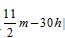, where h is number of hour completed after 12 o’clock and m is the minutes after h hours.

∴ Q = 30 × 7 – 5.5 × 31 = 210 – 170.5 = 39.5°

Now, complementary angle of 39.5° is 90° – 39.5°= 50 .5°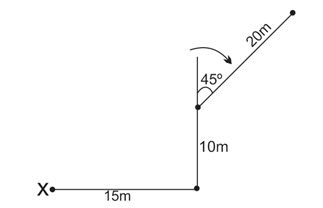3 => 32 + 33 = 9 + 27 = 36

Similarly,

7 =>72 + 73 = 49 + 343 = 392

15 = > Factors of 15 = 1, 3, 5, and 15

Sum of all the factors of 15 = 1 + 3 + 5 + 15 = 24

Similarly, 18 = 1, 2, 3, 6, 9, 18 = 39

So, Sum of factors = 1+ 2+ 3 + 6 + 9 + 18 = 39

Violet is type of colour, Alpha, gama, Beeta is under the rays and its category.

‘Psychology’ is a subject is which we deal with human psychologies and its behavior except it; all the three options are related to geography and its types.

Number of leap years from 1986 to 2016 is ‘8’ total number of odd days from 5th sep. 1986 to 5th December 2016 = 30 + 8 = 38 => 3

Therefore, 5th December 2016 will be Monday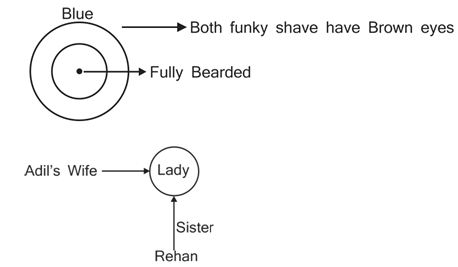Bachelor and Ali have the same colour Eyes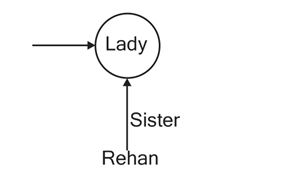Bachelor and Ali have the same colour Eyes, One Bachelor and Ali have Brown eyes and both are funky – shaved.

Ali is married, since Adil is unmarried.

Rehan is not married.

Since, Rehan and Ali are fanky – shaved.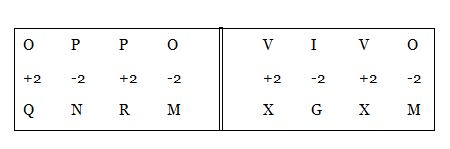*********************************